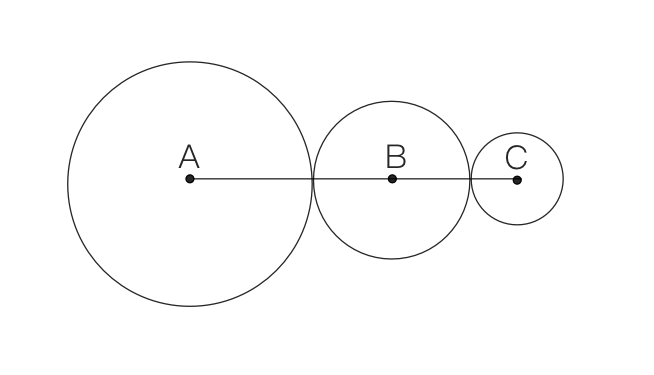### Still have math questions?

Q:

A rectangular region is removed from another rectangular region to create the shaded region shown below. Find the area of the shaded region.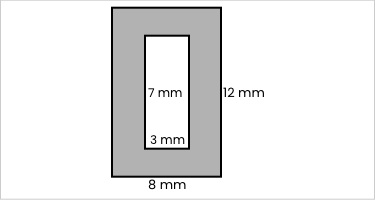Q:

Given $$m\parallel n$$, find the value of x.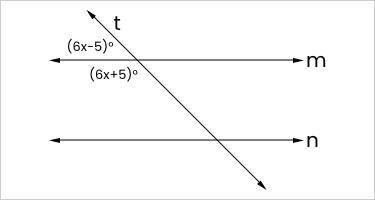Q: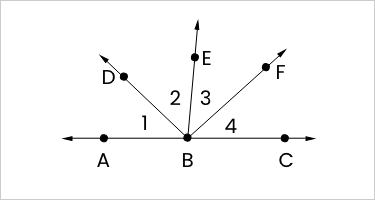In the figure $$\overrightarrow{BA}$$ and $$\overrightarrow{BC}$$ are opposite rays. $$\overrightarrow{BF}$$ bisects $$\angle\text{CBE}$$.

If $$m\angle\text{EBF}=6x+4$$ and $$m\angle\text{CBF}=7x-2$$, find $$m\angle\text{EBF}$$.

Q:

A rectangular athletic field is 100 yards wide and 500 yards long. How many square yards are there in the field?

Q:

Given the points C(-1, -3) and D(5, 6), find the coordinates of the point E on directed line segment CD that partitions CD into the ratio 2 to 1.

Q:

A translation maps the point E(-3, 10) to the point E'(2,1). If the same translation maps point F to the image point F'(7, -4) then which of the following are the coordinates of F?

(1) F(-6, 12)

(2) F(7, 4)

(3) F(12, -13)

(4) F(2, 5)

Q:

The vertices of $$\triangle$$WED are W(5, -2), E(-3, -4), and D(1, 6).

True or false. After dilation with center D and scale factor of 3, the coordinates of the images are W'(15, -6), E'(-9, -12), and D'(3, 18).

Q:

Find the volume of a pyramid with a square base, where the side length is the base is 7.5 cm and the height of the pyramid is 10.2 cm. Round your answer to the nearest tenth of a cubic centimeter.

Q:

Find the perimeter of the composite geometric figure, which consists of three semicircles and a square. Use $$\pi\approx3.14$$.(Round to the nearest hundredth.)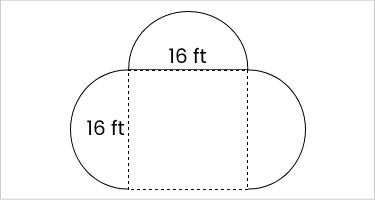Q:

Find the height, x, of the triangle.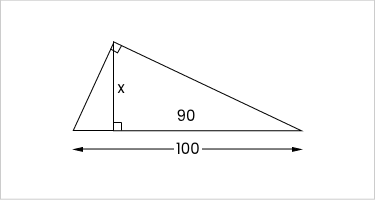A 30

B 40

C 50

D 60

Q:

$$\triangle\text{ABC}$$ and $$\triangle\text{DEF}$$ are congruent. If $$\text{AB}=\text{DE}$$$$\text{BC}=\text{EF}$$$$\text{m}\angle\text{ABC}=37^{\circ}$$, and $$\text{m}\angle\text{EDF}=39^{\circ}$$, what is the measure (in degrees) of $$\angle\text{EFD}$$?

Q:

Find the length of the hypotenuse in the following right triangle.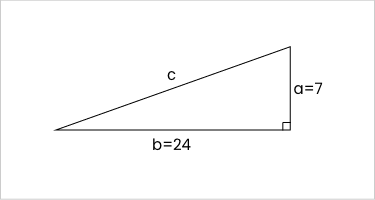Q:

$$\overleftrightarrow{AD}$$ is the perpendicular bisector of $$\overleftrightarrow{BC}$$$$BD=5x-10$$ and $$DC=3x+10$$. Determine the value of x.

Q:

In the figure below, if $$m\angle\text{AXC}=10x$$, then $$m\angle\text{BXD}=?$$.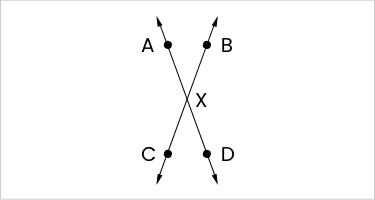$$10x$$

$$360-10x$$

$$90-10x$$

$$180-10x$$

Q:

Triangle LMN is translated to L'M'N'. L was located at -1,3 and L' was found at -8,1. If M is at 1,3 what would be the coordinates of M' be?

Q:

Find an equation of the circle that has center (-6,4) and passes through (-1, -2).

Q:

Find the length of the missing to the nearest tenth(do not forget your units).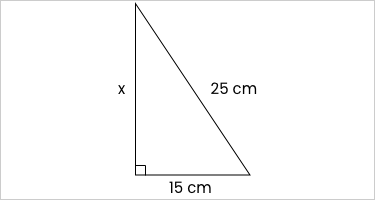Q:

Solve for $$\text{m}\angle\text{PRS}$$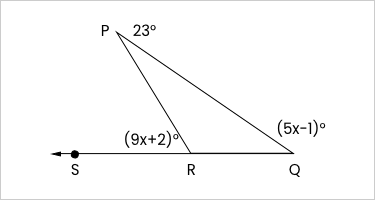$$\angle\text{PRS}=(9x+2)^{\circ}$$

$$\angle\text{PQR}=(5x-1)^{\circ}$$

$$\angle\text{RPQ}=23^{\circ}$$

Q:

Examine the following equation and determine the y-intercept and slope: y=-x

a. Slope is -x and intercept is 0

b. Slope is -x and y intercept is -1

c. Slope is -1 and y intercept is 0

d. Slope is -1 and y intercept is -1

Q:

The circumference of a circle is 16$$\pi$$ cm. What is the area, in square centimeters? Express your answer in terms of $$\pi$$.

Q:

Calculate the area of a circle with a diameter of 10.8 centimeters.

Q:

Which of the following sets of lengths can represent the measures of the sides of a right triangle?

A. 4, 5,6

B. 5, 12, 15

C. 8, 10, 17

D. 20, 21, 29

Q:

In the rectangle, if $$SQ=11x-26$$ and $$PR=5x+28$$, find $$PR$$.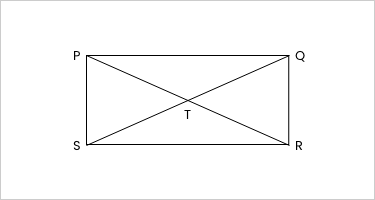Q:

What is the measure of each angle of a regular 18-gon? If necessary, round to the nearest tenth.

Q:

In the figure, the radius of circle A is twice the radius of circle B and four times the radius of circle C. If the sum of the circumferences of the three circles is 42π, find the measure of AC.